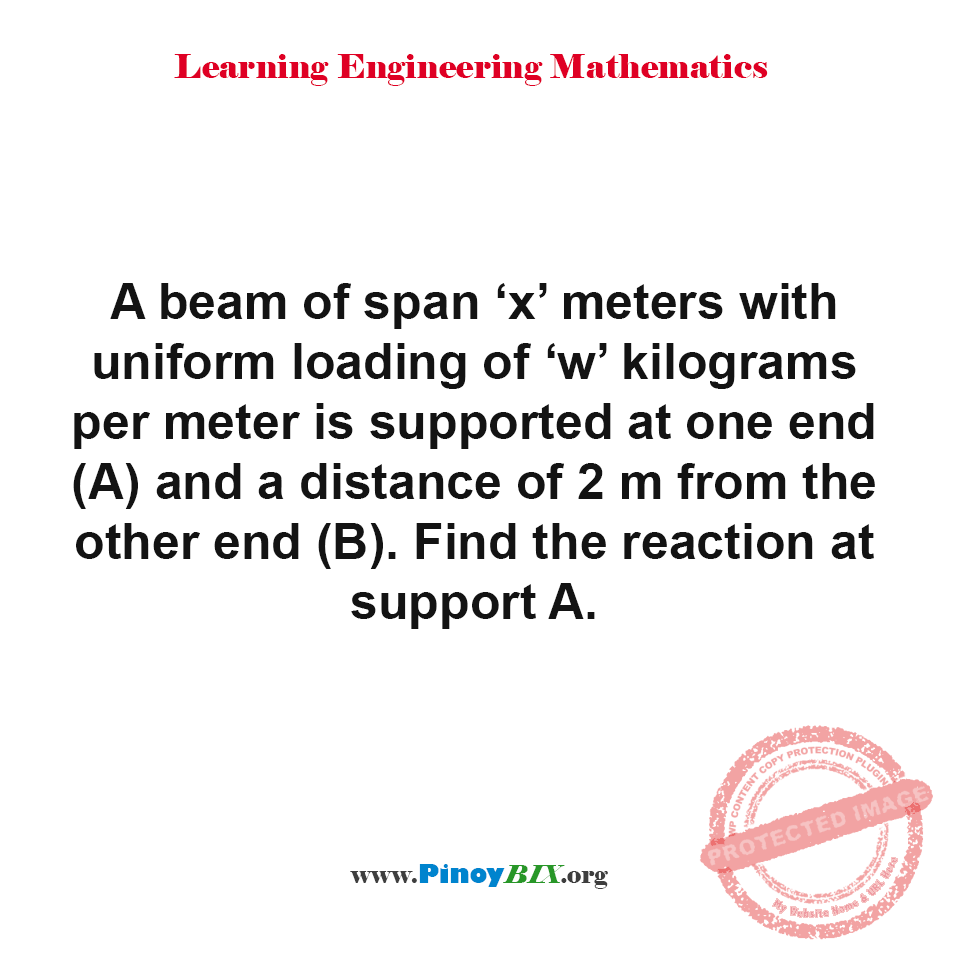# Solution: Find the reaction at support A

(Last Updated On: December 20, 2017)#### Problem Statement:

A beam of span ‘x’ meters with uniform loading of ‘w’ kilograms per meter is supported at one end (A) and a distance of 2 m from the other end (B). Find the reaction at support A.

• A. (wx^2)/ [2(x-2)] kg
• B. [wx(x-4)]/[2(x-2)] kg
• C. [wx(x-2)]/[2(x-2)] kg
• D. wx/[2(x-2)] kg

The reaction at support A is [wx(x-4)]/[2(x-2)] kg.

### Latest Problem Solving in Statics

More Questions in: Engineering Mechanics Statics

Rate this: